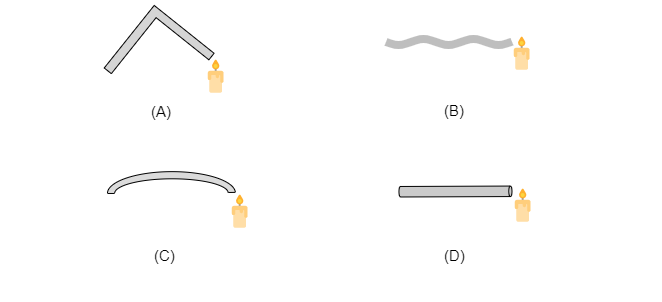Who will be able to see the candle flame clearly?
$(a)$. A
$(b)$. B
$(c)$. C
$(d)$. D" ">

# Four students A, B, C, and D looked through pipes of different shapes to see a candle flame as shown in the figure.Who will be able to see the candle flame clearly?$(a)$. A$(b)$. B$(c)$. C$(d)$. D"

A, B, and C looked through the pipes which are not straight. Only A looked through the straight pipe and we know that light travels only in a straight line.

So, option $(d)$ is correct.

Updated on: 10-Oct-2022

16 Views